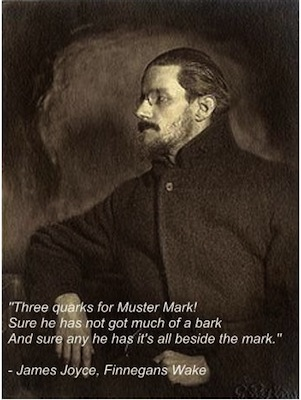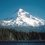# Three Quarks For You!Murray Gell-Mann, one of the inventors of the quark model, used to ocassionally peruse the novel Finnegans Wake, by James Joyce (pictured above). When naming the particle he discovered, Gell-Mann had the sound he wanted, "kwork," but not the spelling. He chose to spell it quark after reading the above passage from Finnegans Wake.

In this post, we present a worked solution to our recent problem of the week. A list of students who submitted correct solutions can be found here. These students were entered into a drawing to win a free Brilliant.org t-shirt. To recap, the problem was:

## Three quarks for you!

Neutrons and protons, which make up the nuclei of atoms, are themselves made up of smaller particles called quarks. A proton is made of two "up" quarks and one "down" quark while a neutron is made of two down quarks and one up quark. Quarks have an interesting property called confinement, in that no single quark can be isolated and observed. We can still measure their properties such as mass in accelerators, but there's a simple way to get an estimate of mass differences between quarks using elementary electromagnetism and the masses of the neutron and proton. How much heavier is the down quark than the up quark in kilograms, if the the mass difference between the neutron and proton is due to the different total quark masses and the different electrostatic energy?

To convert energy into mass and vice versa, use $E=mc^{2}$.

### Details and assumptions

1. The neutron is heavier than the proton by $2.306\times10^{-30}kg$.
2. The electrostatic constant is $K=9 \times10^{9} \frac{Nm^{2}}{C^{2}}$.
3. The speed of light is $3\times10^{8} \frac{m}{s}$.
4. The down quark has a charge $q_d=\frac{e}{3}$ and the up quark has a charge $q_u=\frac{-2e}{3}$, where e is the charge on an electron, $e=-1.602\times10^{-30}$ Coulombs.
5. Quarks can be treated as point charges and the distance between quarks in a proton or neutron is about $r=10^{-15}$.
6. The real answer is around 2 to $3\times10^{-30}$, so as you will see we're going to get pretty close.

### Solution:

First, let's define $q_u=Ue$ and $q_d=De$ for the charge of the up and down quark, respectively. Since we can treat the quarks as point charges, the electrostatic energy is just the usual electrostatic potential energy, for example between two point charges $E=\frac{Kq_1q_2}{r}$. For the three charges in the proton, the total electrostatic energy is

$E_p=Ke^2\frac{(U^2+2UD)}{r}=0$

while for the neutron it's

$E_n=Ke^2\frac{(D^2+2UD)}{r}=\frac{-Ke^2}{3r}=-7.7 \times 10^{-14}~J.$

The difference between the neutron and proton mass will give us the difference between the down quark and up quark mass. With a little linear algebra and $E=mc^2$ to convert energy into mass, we have

$m_n-m_p=((2m_d+m_u)+\frac{E_n}{c^2})-((2m_u+m_d)+\frac{E_p}{c^2}) .$

This implies that

$m_d-m_u=(m_n-m_p)-\frac{E_n-E_p}{c^2}=3.16 \times 10^{-30}~kg.$

Now, the astute problem solver might ask why in the world we'd ask for the difference in quark masses. Wouldn't a more interesting problem be to actually find the quark masses themselves from the neutron and proton mass? We could have done that, but while the difference between the masses would remain correct, the actual masses calculated for the quarks would have been way off. This is because the picture of a proton or neutron being made up of just three quarks is, well, a little incorrect. There is actually a whole lot more to a proton and neutron. But, by asking about the difference in the masses we can construct a problem that gets pretty close to the right value in the real world as the "whole lot more" roughly cancels out.Note by Peter Taylor
6 years, 6 months ago

This discussion board is a place to discuss our Daily Challenges and the math and science related to those challenges. Explanations are more than just a solution — they should explain the steps and thinking strategies that you used to obtain the solution. Comments should further the discussion of math and science.

When posting on Brilliant:

• Use the emojis to react to an explanation, whether you're congratulating a job well done , or just really confused .
• Ask specific questions about the challenge or the steps in somebody's explanation. Well-posed questions can add a lot to the discussion, but posting "I don't understand!" doesn't help anyone.
• Try to contribute something new to the discussion, whether it is an extension, generalization or other idea related to the challenge.

MarkdownAppears as
*italics* or _italics_ italics
**bold** or __bold__ bold
- bulleted- list
• bulleted
• list
1. numbered2. list
1. numbered
2. list
Note: you must add a full line of space before and after lists for them to show up correctly
paragraph 1paragraph 2

paragraph 1

paragraph 2

[example link](https://brilliant.org)example link
> This is a quote
This is a quote
    # I indented these lines
# 4 spaces, and now they show
# up as a code block.

print "hello world"
# I indented these lines
# 4 spaces, and now they show
# up as a code block.

print "hello world"
MathAppears as
Remember to wrap math in $$ ... $$ or $ ... $ to ensure proper formatting.
2 \times 3 $2 \times 3$
2^{34} $2^{34}$
a_{i-1} $a_{i-1}$
\frac{2}{3} $\frac{2}{3}$
\sqrt{2} $\sqrt{2}$
\sum_{i=1}^3 $\sum_{i=1}^3$
\sin \theta $\sin \theta$
\boxed{123} $\boxed{123}$